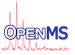OpenMS
RANSAC< TModelType > Class Template Reference

This class provides a generic implementation of the RANSAC outlier detection algorithm. Is implemented and tested after the SciPy reference: http://wiki.scipy.org/Cookbook/RANSAC. More...

#include <OpenMS/MATH/MISC/RANSAC.h>

Collaboration diagram for RANSAC< TModelType >:
[legend]

## Public Member Functions

RANSAC (uint64_t seed=time(nullptr))

~RANSAC ()=default

void setSeed (uint64_t seed)
set seed for random shuffle More...

std::vector< std::pair< double, double > > ransac (const std::vector< std::pair< double, double > > &pairs, const RANSACParam &p)
alias for ransac() with full params More...

std::vector< std::pair< double, double > > ransac (const std::vector< std::pair< double, double > > &pairs, size_t n, size_t k, double t, size_t d, bool relative_d=false)
This function provides a generic implementation of the RANSAC outlier detection algorithm. Is implemented and tested after the SciPy reference: http://wiki.scipy.org/Cookbook/RANSAC. More...

## Private Attributes

Math::RandomShuffler shuffler_ {}

## Detailed Description

### template<typename TModelType = RansacModelLinear> class OpenMS::Math::RANSAC< TModelType >

This class provides a generic implementation of the RANSAC outlier detection algorithm. Is implemented and tested after the SciPy reference: http://wiki.scipy.org/Cookbook/RANSAC.

## ◆ RANSAC()

 RANSAC ( uint64_t seed = time(nullptr) )
inlineexplicit

## ◆ ~RANSAC()

 ~RANSAC ( )
default

## ◆ ransac() [1/2]

 std::vector > ransac ( const std::vector< std::pair< double, double > > & pairs, const RANSACParam & p )
inline

alias for ransac() with full params

## ◆ ransac() [2/2]

 std::vector > ransac ( const std::vector< std::pair< double, double > > & pairs, size_t n, size_t k, double t, size_t d, bool relative_d = false )
inline

This function provides a generic implementation of the RANSAC outlier detection algorithm. Is implemented and tested after the SciPy reference: http://wiki.scipy.org/Cookbook/RANSAC.

If possible, restrict 'n' to the minimal number of points which the model requires to make a fit, i.e. n=2 for linear, n=3 for quadratic. Any higher number will result in increasing the chance of including an outlier, hence a lost iteration.

While iterating, this RANSAC implementation will consider any model which explains more data points than the currently best model as even better. If the data points are equal, RSS (residual sum of squared error) will be used.

Making 'd' a relative measure (1-99%) is useful if you cannot predict how many points RANSAC will actually receive at runtime, but you have a rough idea how many percent will be outliers. E.g. if you expect 20% outliers, then setting d=60, relative_d=true (i.e. 60% inliers with some margin for error) is a good bet for a larger input set. (Consider that 2-3 data points will be used for the initial model already – they cannot possibly become inliers).

Parameters
 pairs Input data (paired data of type ) n The minimum number of data points required to fit the model k The maximum number of iterations allowed in the algorithm t Threshold value for determining when a data point fits a model. Corresponds to the maximal squared deviation in units of the _second_ dimension (dim2). d The number of close data values (according to 't') required to assert that a model fits well to data relative_d Should 'd' be interpreted as percentages (0-100) of data input size rng Custom RNG function (useful for testing with fixed seeds)
Returns
A vector of pairs fitting the model well; data will be unsorted

## ◆ setSeed()

 void setSeed ( uint64_t seed )
inline

set seed for random shuffle

References RandomShuffler::seed(), and RANSAC< TModelType >::shuffler_.

## ◆ shuffler_

 Math::RandomShuffler shuffler_ {}
private